Courses

# AC Bridges to Electrical & Electronics Measurements

## 10 Questions MCQ Test Topicwise Question Bank for Electronics Engineering | AC Bridges to Electrical & Electronics Measurements

Description
This mock test of AC Bridges to Electrical & Electronics Measurements for Electrical Engineering (EE) helps you for every Electrical Engineering (EE) entrance exam. This contains 10 Multiple Choice Questions for Electrical Engineering (EE) AC Bridges to Electrical & Electronics Measurements (mcq) to study with solutions a complete question bank. The solved questions answers in this AC Bridges to Electrical & Electronics Measurements quiz give you a good mix of easy questions and tough questions. Electrical Engineering (EE) students definitely take this AC Bridges to Electrical & Electronics Measurements exercise for a better result in the exam. You can find other AC Bridges to Electrical & Electronics Measurements extra questions, long questions & short questions for Electrical Engineering (EE) on EduRev as well by searching above.
QUESTION: 1

### A bridge circuit works at a frequency of 3 kHz. Which detector can be used for detecting the null conditions in the bridge?​

Solution:

For 3 kHz frequency headphone (Range - 250 Hz to 4 kHz) and tuned amplifier detector (Range - 10 Hz to 100 kHz) will be used.

QUESTION: 2

Solution:
QUESTION: 3

### The equations under conditions of balance for a bridge measuring resistance R1, and inductance L1 of a choke coil are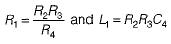In order to achieve convergence of balance

Solution:

The bridge circuit for the given balance condition indicates that of a Maxwell’s inductance capacitance bridge as shown below.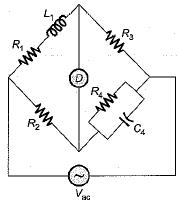For sliding balance, R4 and Cwill be varied since they are not common in the equations of R1, and L1.
in order to achieve convergence to balance point, R4 and C4 will be varied since they are in the same arm of the bridge.

QUESTION: 4

In measurement of resistance by “Carey Foster bridge” no error is introduced due to

Solution:

“Carey Foster slide wire bridge” is a special type of bridge circuit which is used for the measurement of medium resistance by comparing with a standard known resistance. This bridge circuit will give no error due to connecting lead resistances, thermoelectric emfs and contact resistances.

QUESTION: 5

A capacitance comparison bridge as shown in figure below is used to measure a capacitive impedance at a frequency of 2 kHz. The bridge constants at balance are: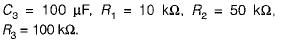The equivalent series circuit of the unknown impedance will be represented as:

Solution:

At balance condition,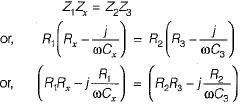Separating real and imaginary parts, we have: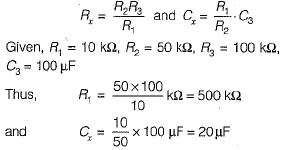QUESTION: 6

A Wien bridge circuit consists of the following: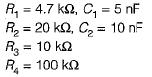The frequency of the circuit is

Solution:

The frequency measured by the Wien bridge is:QUESTION: 7

An ac bridge uses a detector in the form of

Solution:
QUESTION: 8

Maxwell’s bridge is used to measure Q-factor in the range

Solution:

Maxwell’s bridge is used to measure Q-factor of medium Q-coils i.e. 1 < Q < 10

QUESTION: 9

Anderson bridge is used to measure unknown

Solution:

Anderson bridge is used for the measurement of low Q -coils i.e. measurement of low inductance.

QUESTION: 10

Hay’s bridge is used to measure an inductance of

Solution:

Hay’s bridge is used for the measurement of inductance of high Q-coils (Q > 10).